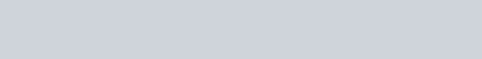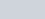Find the mean of the following frequency distribution,
Question:

Find the mean of the following frequency distribution, using step-deviation method:Solution:

The following frequency distribution is givenWe have to find the mean of the above frequency distribution using step deviation method.

By using step deviation method, we haveFrom the above distribution, we have

$h=10$

$a=25$

$\sum f_{i}=50$

$\sum f_{i} u_{i}=0$

We know the mean of a given distribution is given by

$\operatorname{Mean}(\bar{x})=a+\frac{\sum f_{t} u_{i}}{\sum f_{i}} \times h$

$=25+\frac{0}{50} \times 10$

$=25$

Hence the mean of the given distribution is 25.